Filter search results
Sort by
Content type
Image orientation
Color composition
People
Number of people
Gender
Age group
Ethnicity
More filters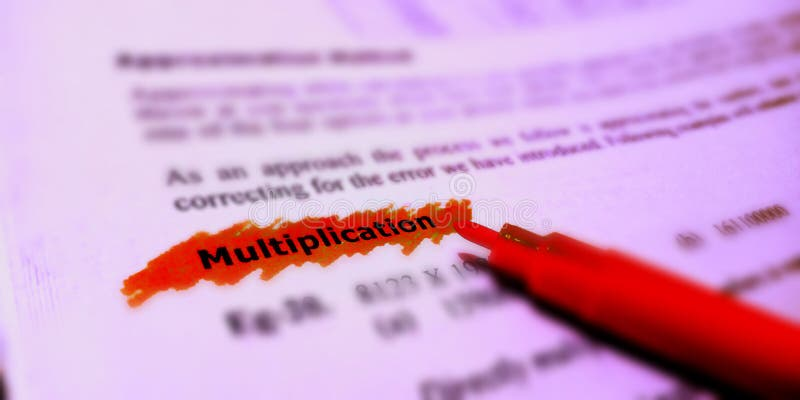Multiplication text written on english language with red colour covering text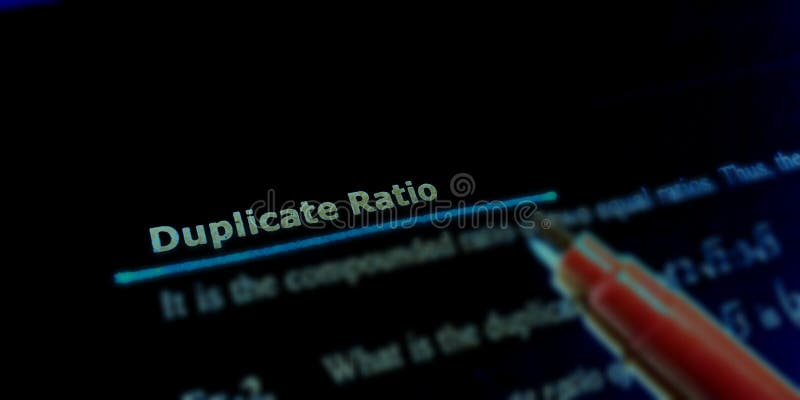Duplicate ratio mathematical words displaying at digital black background illustrationsNatural soil text hand written text on horizontal land concept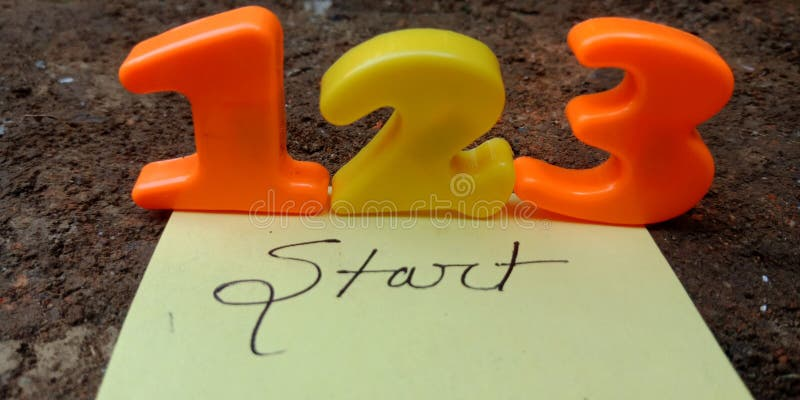123 start kids exam concept on horizontal land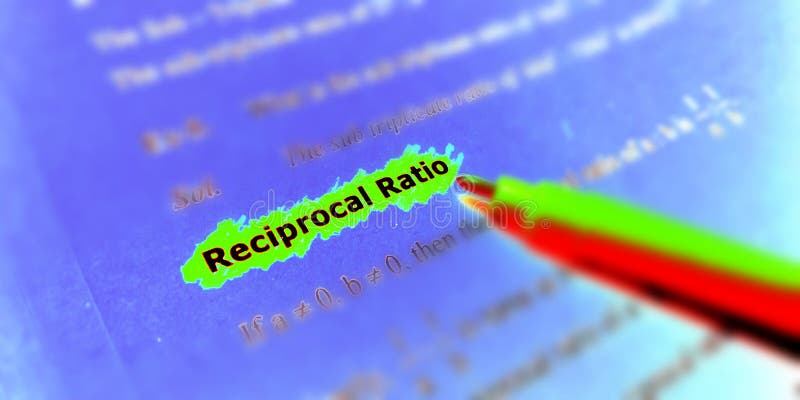Reciprocal ratio text highlighted on green colour highlighted background illustrations image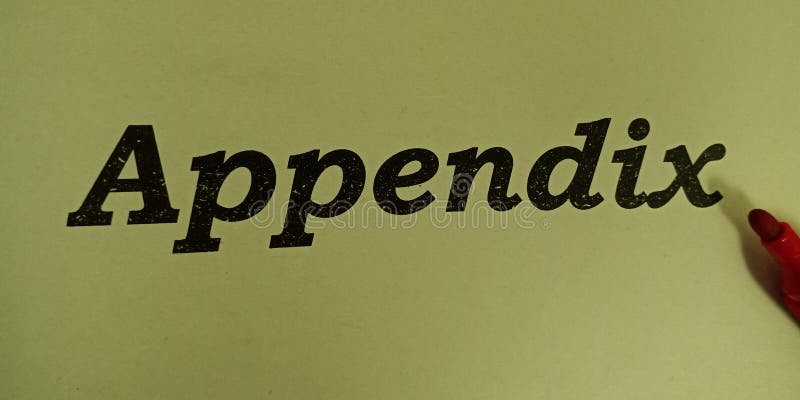Appendix words in black english letters written on paper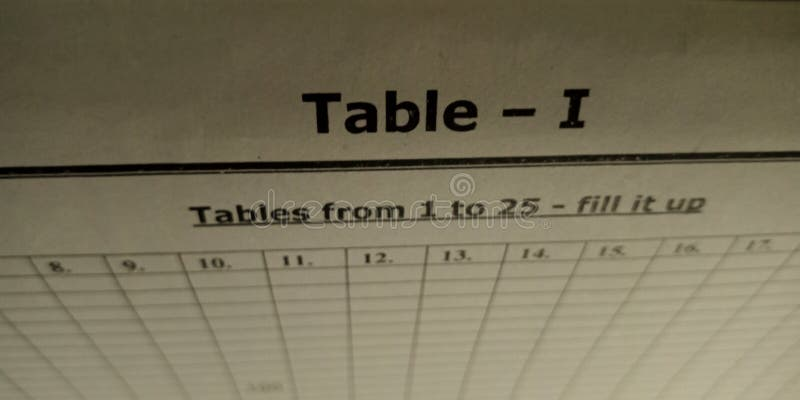Table one text presenting on white paper sheet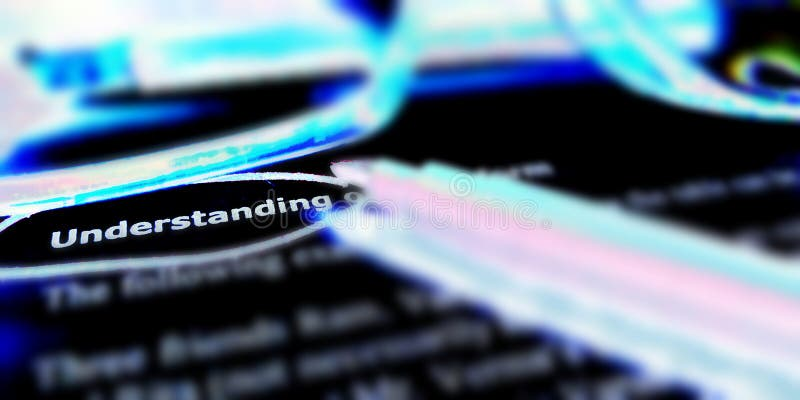Understanding text highlighted on illustrations image with blue black background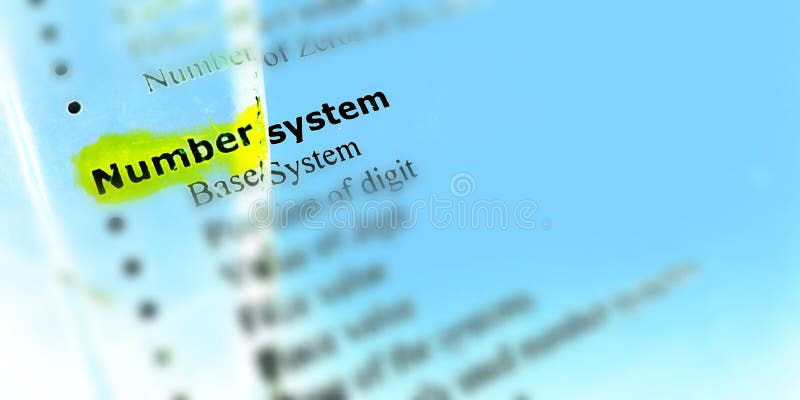Number system words highlighted in yellow color on glass image. Start, kids, exam, concept, horizontal, land, ratio, text, green, colour, background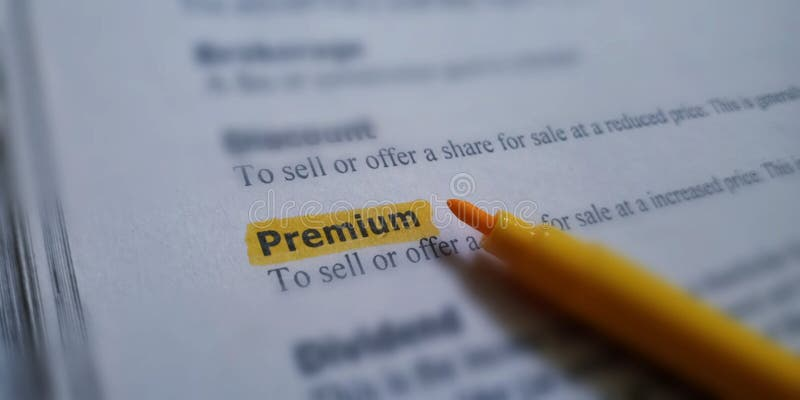Premium english text written on english language with yellow highlighted patternInvestment Chart with glasses and computer. Investment Chart, glasses and computerInvestment Chart with glasses. FinancesMontessori panel with mathematical operations to learn multiplication tables in a school.Profit earned line graph presentation on white paper sheet with green highlightedFuture text written behind the specs on horizontal land backgroundBar and table word with red pencil on white paperRanking numerical series presenting on white background with yellow sketch penMulti-colored multiplication table in the vector. Located on a green background with a school pattern. Poster for kids. Times. Table. Help to learn mathematicsPile of coins with a chart in the background. A pile of Euro Cent coins with a Euro Chart in the backgroundMontessori panel with mathematical operations to learn multiplication tables in a school.Montessori panel with mathematical operations to learn multiplication tables in a school.Montessori panel with mathematical operations to learn multiplication tables in a school.Montessori panel with mathematical operations to learn multiplication tables in a school.Montessori panel with mathematical operations to learn multiplication tables in a school.Multiplication Table. Multiplication or times table showing zero to ten multiplesA chart of twelve stacks of gold coins. A chart of twelve stacks of gold coloured euro coinsConsumption mathematical reasoning text highlighted on white paper sheet. Consumption mathematical reasoning text highed on white paper sheet, , earned, lineClose up. the blurred image of the multiplication table on white. BackgroundSleeping partner text written on red colour text highlighted pattern on white sheet. School, engineering, colored, numerical, series, ing, background, yellowClose up. the blurred image of the multiplication table on white. BackgroundClose up. the blurred image of the multiplication table on white. BackgroundSchool of engineering text written in red colored textPie charts text underlined with red colour on white paperClose up. the blurred image of the multiplication table on white. BackgroundSinking fund word in english language on illustrations imageTarget looking into specs glass around soil background. Pie, charts, text, underlined, red, colour, white, paper, ratio, mathematical, words, displayingProblems on red green signal concept white paperFinancial employees are working on investments. Brokers work in the office. Investments, multiplication of profits, finances conceptMan in front of charts. Young business man standing in front of some graphs and charts with a pensive look on his faceMouse and calculator. Vertical image of mouse and calculatorPrepare purchasing and logistics. Arrangement of office supply, calculator, logistics signs and blocks closeupCalculator & Charts. Detail closeup of a calculator on a desk topInvestment Graph with glasses. FinancesFinances and balances with pen. Counting finances and balances with penMagnifier lies on the financial charts in the office. Black and white backgroundMagnifier lies on the financial charts in the office. Black and white backgroundMathematics formula on blackboard. Mathematics formula of geometric shape written on blackboardA close-up of golden fifty cent coins. Against a rind backgroundEducation mathematics concept. With a surprised boyArithmetics. Several complex mathematical formulas, equations, and geometry written on a smooth black boardMathematics formula on blackboard. Mathematics formula of geometric shape written on blackboardComposite image of portrait of businessman yawning. Portrait of businessman yawning against grey vignetteStudent writing math on whiteboard. A student writes several complex mathematical formulas, equations, and geometry on a white board. The point of focus is onStudent writing math on whiteboard. A student writes several complex mathematical formulas, equations, and geometry on a white board. The point of focus is onComposite image of businessman standing back to camera writing with marker. Businessman standing back to camera writing with marker against grey roomComposite image of businessman standing back to camera writing with marker. Businessman standing back to camera writing with marker against grey roomCalculating finances. Calculator and pen on business graphicsCalculating finances. Calculator and pen on business graphicsTrapezoid area formula. Written on blackboardCuboid. Technical chalkboard white mathematics formula countTriangle area formula. Written on blackboardCircle area and perimeter formula. Written on blackboardMathematics. Several complex mathematical formulas, equations, and geometry written on a smooth black boardFinancial employees are working on investments. Brokers work in the office. Investments, multiplication of profits, finances conceptGeometry. Several complex mathematical formulas, equations, and geometry written on a smooth black boardGeometry. Several complex mathematical formulas, equations, and geometry written on a white boardAbstract math background. Several complex mathematical formulas, equations, and geometry written on a white boardComplex Mathematics background. Several complex mathematical formulas, equations, and geometry written on a smooth black boardAutomatic pen, calculator, notebook on a white background close-up.Composite image of mid section of businessman writing with marker. Mid section of businessman writing with marker against grey backgroundFinancial employees are working on investments. Brokers work in the office. Investments, multiplication of profits, finances conceptCuboid volume formula. Written on blackboardMathematics subject. Back to School background. Education banner. Mathematics subject. Back to School background. Education bannerSpeed of light. Derrivation equation and formulasCalculator with different symbols around and a pen on the side. Calculator on a white background with different symbols all around and a pencil on the sideMathematician demonstrate how to determine the area of a circle. Mathematical equation to illustrate the use of a formula to show the area of a circleMathematician demonstrate how to determine the area of a circle. Mathematical equation to illustrate the use of a formula to show the area of a circleMathematician demonstrate how to determine the area of a circle. Mathematical equation to illustrate the use of a formula to show the area of a circleOffice equipment. The very important office equipmentMathematics subject. Back to School background. Education banner. Mathematics subject. Back to School background. Education banner